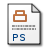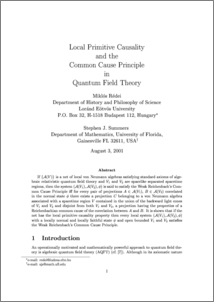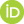# Local primitive causality and the common cause principle in quantum field theory

Redei, Miklos and Summers, Stephen J. (2001) Local primitive causality and the common cause principle in quantum field theory. [Preprint]Preview
Postscript
qftcause.ps

## Abstract

If $\{{\cal A}(V)\}$ is a net of local von Neumann algebras satisfying standard axioms of algebraic relativistic quantum field theory and $V_1$ and $V_2$ are spacelike separated spacetime regions, then the system $({\cal A}(V_1),{\cal A}(V_2),\phi)$ is said to satisfy the Weak Reichenbach's Common Cause Principle iff for every pair of projections $A\in{\cal A}(V_1)$, $B\in{\cal A}(V_2)$ correlated in the normal state $\phi$ there exists a projection $C$ belonging to a von Neumann algebra associated with a spacetime region $V$ contained in the union of the backward light cones of $V_1$ and $V_2$ and disjoint from both $V_1$ and $V_2$, a projection having the properties of a Reichenbachian common cause of the correlation between $A$ and $B$. It is shown that if the net has the local primitive causality property then every local system $({\cal A}(V_1),{\cal A}(V_2),\phi)$ with a locally normal and locally faithful state $\phi$ and open bounded $V_1$ and $V_2$ satisfies the Weak Reichenbach's Common Cause Principle.

 Export/Citation: EndNote | BibTeX | Dublin Core | ASCII/Text Citation (Chicago) | HTML Citation | OpenURL
 Social Networking:

Item Type: Preprint
Creators:
CreatorsEmailORCIDRedei, Miklos
Summers, Stephen J.
Keywords: Common cause, quantum field theory
Subjects: Specific Sciences > Physics > Quantum Field Theory
Depositing User: Miklos Redei
Date Deposited: 08 Aug 2001
Item ID: 352
Subjects: Specific Sciences > Physics > Quantum Field Theory
Date: August 2001
URI: http://philsci-archive.pitt.edu/id/eprint/352

### Monthly Views for the past 3 yearsView Item